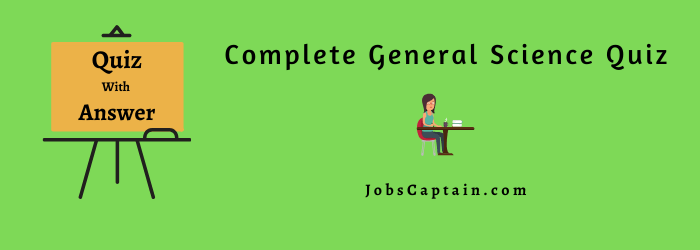# General Science QuizAll quizzes on General Science with answers also you can get pdf. You are welcome to this quick General Science quiz. It has twenty basic questions about various general knowledge and economies. So, let’s get started.

As you know the General Science Questions answers are a very useful subject for all class students and TET, UPSC IAS, State PCS, CTET, SSC, Banking, and for various Competitive Exams.

## Complete General Science Quiz with Answers

Question: The earth satellite Aryabhatta was put in orbit by India on __________.

(A) October 20, 1978

(B) November 14, 1978

(C) January 26, 1979

(D) April 19, 1975

(D) April 19, 1975

Question: The instrument used to measure atmospheric pressure is ___________.

(A) Pyrometer

(B) Eudiometer

(C) Barometer

(D) Hydrometer

(C) Barometer

Question: A light year means ___________.

(A) a unit of length

(B) a unit of time

(C) a year of no consequence

(D) illumination throughout the year

(A) a unit of length

Question: The sun continuously produces an enormous amount of energy. This is due to ___________.

(A) nuclear fission

(B) nuclear fusion

(C) chemical combustion

(D) boiling

(B) nuclear fusion

Question: The principle on which a Jet engine works is __________.

(A) conservation of mass

(B) Newtonian law of action and reaction

(C) conservation of angular momentum

(D) None of the above

(B) Newtonian law of action and reaction

Question: A man Jumping out of a moving train is thrown ____________.

(A) forward

(B) backward

(C) sideways

(D) None of the above

(A) forward

Question: It is easier to climb down a staircase than climb up because in climbing down __________.

(A) work is done against gravity

(B) the steepness of the staircase isn’t felt

(C) gravity assists

(D) there is no friction between the legs and steps

(C) gravity assists

Question: In which of the following will a piece of iron weigh most?

(A) in air

(B) in an atmosphere of oxygen

(C) in an atmosphere of CO2

(D) in vacuum

(D) in vacuum

Question: The unit of power in SI is ____________.

(A) Joule

(B) Newton

(C) Joule/Sec

(D) Erg/Sec

(C) Joule/Sec

Question: Chronometer is an instrument to measure _____________.

(A) heat

(B) electric potential

(C) time

(D) distance between two points

(C) time

Question: Which physical quantity is measured in watts _____________.

(A) power

(B) kinetic energy

(C) momentum

(D) impulse

(A) power

Question: When the velocity of a body is halved ______________.

(A) its momentum is halved

(B) its kinetic energy is halved

(C) its acceleration is halved

(D) its potential energy is halved

(A) its momentum is halved

Question: Mercury is used in thermometers because _________________.

(A) it has a high thermal conductivity

(B) it has uniform expansivity

(C) it has a high boiling point and low freezing point

(D) all the above

(D) all the above

Question: An astronomical unit of distance is _______.

(A) a kilometer

(B) light year

(C) the average distance from the earth to the sun

(D) None of the above

(B) light year

Question: The barometer was invented by ______________.

(B) Torricelli

(C) Thomas Alva Edison

(D) Benjamin Franklin

(B) Torricelli

Question: Where does the center of gravity of a rubber ring lie?

(A) on the outer surface

(B) in the inner surface

(C) at the centre of the ring

(D) None of the above

(C) at the centre of the ring

Question: A temperature below absolute zero for an ideal gas __________.

(A) cannot be attained experimentally

(B) can be attained

(C) is impossible

(D) Does not exist at all

(C) is impossible

Question: The atmosphere gets heated up mainly by ____________.

(A) direct sun’s rays

(D) cosmic rays coming from space

Question: The hygrometer is an apparatus used for finding the _____________.

(A) the density of a liquid

(B) relative humidity of the atmosphere

(C) intensity of light

(D) temperature of air

(B) relative humidity of the atmosphere

Question: In a refrigerator, the refrigerant is _________________.

(A) Nitrogen

(B) Oxygen

(C) Chlorine

(D) Freon